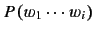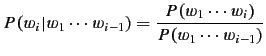Next: Analysis Up: Estimation Algorithm Previous: Estimation Algorithm

## Word Prediction

So far, we have discussed smoothing a probability mass using Simple Good-Turing. After computing smooth mass values, we compute the following using them. Our word prediction is associated with thethat maximizes the following equation.(2)

jac 2010-05-11